עזרה להגן על שונית המחסום הגדולה עם TensorFlow על Kaggle

# שידורי וידאו תוך שימוש במפות 3D

יונפנג לי, דומיניק רובלק ומרקו טגליאסאצ'י. מכאן לשם: סרטון בין לבין באמצעות פיתולי תלת מימד ישירים, 2019.

מאפייני רכזת נוכחיים:

• יש דגמים לסרטוני דחיפה של רובוט BAIR ומערך נתונים של וידאו פעולה של KTH (אם כי קולאב זה משתמש רק ב-BAIR)
• מערך הנתונים של BAIR כבר זמין ב- Hub. עם זאת, סרטוני KTH צריכים להיות מסופקים על ידי המשתמשים עצמם.
• הערכה בלבד (יצירת וידאו) לעת עתה
• גודל אצווה וגודל מסגרת מקודדים קשה

## להכין

מאז tfds.load('bair_robot_pushing_small', split='test') היה ולהוריד ארכיון 30GB כי מכיל גם את נתוני האימון, אנו ולהוריד ארכיון נפרד המכיל רק את נתוני הבדיקה 190MB. בסיס הנתונים בשימוש כבר בהוצאת העיתון הזה והוא מורשה כבעלי רשיון Creative Commons BY 4.0.

import tensorflow as tf

import matplotlib.pyplot as plt
import numpy as np
import seaborn as sns
import tensorflow_hub as hub
import tensorflow_datasets as tfds

from tensorflow_datasets.core import SplitGenerator
from tensorflow_datasets.video.bair_robot_pushing import BairRobotPushingSmall

import tempfile
import pathlib

TEST_DIR = pathlib.Path(tempfile.mkdtemp()) / "bair_robot_pushing_small/softmotion30_44k/test/"

# Download the test split to $TEST_DIR mkdir -p$TEST_DIR
wget -nv https://storage.googleapis.com/download.tensorflow.org/data/bair_test_traj_0_to_255.tfrecords -O \$TEST_DIR/traj_0_to_255.tfrecords

2021-11-05 12:44:33 URL:https://storage.googleapis.com/download.tensorflow.org/data/bair_test_traj_0_to_255.tfrecords [189852160/189852160] -> "/tmp/tmpn_2q0lmh/bair_robot_pushing_small/softmotion30_44k/test/traj_0_to_255.tfrecords" 

# Since the dataset builder expects the train and test split to be downloaded,
# patch it so it only expects the test data to be available
builder = BairRobotPushingSmall()
test_generator = SplitGenerator(name='test', gen_kwargs={"filedir": str(TEST_DIR)})
builder._split_generators = lambda _: [test_generator]


## BAIR: הדגמה המבוססת על כניסות מערך numpy

Test videos shape [batch_size, start/end frame, height, width, num_channels]:  (16, 2, 64, 64, 3)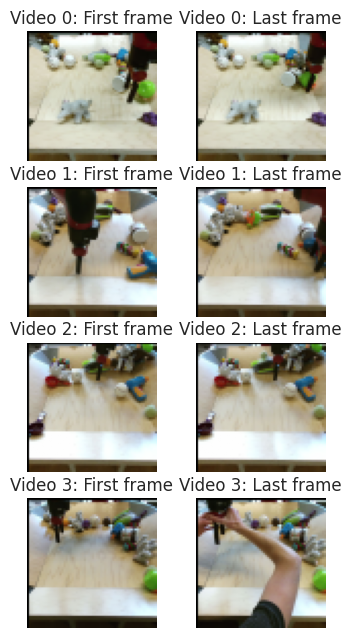### טען את מודול הרכזת

hub_handle = 'https://tfhub.dev/google/tweening_conv3d_bair/1'

WARNING:tensorflow:Unable to create a python object for variable <tf.Variable 'video_discriminator/conv_0/weights:0' shape=(4, 4, 4, 3, 64) dtype=float32_ref> because it is a reference variable. It may not be visible to training APIs. If this is a problem, consider rebuilding the SavedModel after running tf.compat.v1.enable_resource_variables().
WARNING:tensorflow:Unable to create a python object for variable <tf.Variable 'video_discriminator/conv_0/weights:0' shape=(4, 4, 4, 3, 64) dtype=float32_ref> because it is a reference variable. It may not be visible to training APIs. If this is a problem, consider rebuilding the SavedModel after running tf.compat.v1.enable_resource_variables().
WARNING:tensorflow:Unable to create a python object for variable <tf.Variable 'video_discriminator/conv_0/LayerNorm/beta:0' shape=(64,) dtype=float32_ref> because it is a reference variable. It may not be visible to training APIs. If this is a problem, consider rebuilding the SavedModel after running tf.compat.v1.enable_resource_variables().
WARNING:tensorflow:Unable to create a python object for variable <tf.Variable 'video_discriminator/conv_0/LayerNorm/beta:0' shape=(64,) dtype=float32_ref> because it is a reference variable. It may not be visible to training APIs. If this is a problem, consider rebuilding the SavedModel after running tf.compat.v1.enable_resource_variables().
WARNING:tensorflow:Unable to create a python object for variable <tf.Variable 'video_discriminator/conv_0/LayerNorm/gamma:0' shape=(64,) dtype=float32_ref> because it is a reference variable. It may not be visible to training APIs. If this is a problem, consider rebuilding the SavedModel after running tf.compat.v1.enable_resource_variables().
WARNING:tensorflow:Unable to create a python object for variable <tf.Variable 'video_discriminator/conv_0/LayerNorm/gamma:0' shape=(64,) dtype=float32_ref> because it is a reference variable. It may not be visible to training APIs. If this is a problem, consider rebuilding the SavedModel after running tf.compat.v1.enable_resource_variables().
WARNING:tensorflow:Unable to create a python object for variable <tf.Variable 'video_discriminator/conv_1/weights:0' shape=(4, 4, 4, 64, 128) dtype=float32_ref> because it is a reference variable. It may not be visible to training APIs. If this is a problem, consider rebuilding the SavedModel after running tf.compat.v1.enable_resource_variables().
WARNING:tensorflow:Unable to create a python object for variable <tf.Variable 'video_discriminator/conv_1/weights:0' shape=(4, 4, 4, 64, 128) dtype=float32_ref> because it is a reference variable. It may not be visible to training APIs. If this is a problem, consider rebuilding the SavedModel after running tf.compat.v1.enable_resource_variables().
WARNING:tensorflow:Unable to create a python object for variable <tf.Variable 'video_discriminator/conv_1/LayerNorm/beta:0' shape=(128,) dtype=float32_ref> because it is a reference variable. It may not be visible to training APIs. If this is a problem, consider rebuilding the SavedModel after running tf.compat.v1.enable_resource_variables().
WARNING:tensorflow:Unable to create a python object for variable <tf.Variable 'video_discriminator/conv_1/LayerNorm/beta:0' shape=(128,) dtype=float32_ref> because it is a reference variable. It may not be visible to training APIs. If this is a problem, consider rebuilding the SavedModel after running tf.compat.v1.enable_resource_variables().
WARNING:tensorflow:Unable to create a python object for variable <tf.Variable 'video_discriminator/conv_0/weights:0' shape=(4, 4, 4, 3, 64) dtype=float32_ref> because it is a reference variable. It may not be visible to training APIs. If this is a problem, consider rebuilding the SavedModel after running tf.compat.v1.enable_resource_variables().
WARNING:tensorflow:Unable to create a python object for variable <tf.Variable 'video_discriminator/conv_0/weights:0' shape=(4, 4, 4, 3, 64) dtype=float32_ref> because it is a reference variable. It may not be visible to training APIs. If this is a problem, consider rebuilding the SavedModel after running tf.compat.v1.enable_resource_variables().
WARNING:tensorflow:Unable to create a python object for variable <tf.Variable 'video_discriminator/conv_0/LayerNorm/beta:0' shape=(64,) dtype=float32_ref> because it is a reference variable. It may not be visible to training APIs. If this is a problem, consider rebuilding the SavedModel after running tf.compat.v1.enable_resource_variables().
WARNING:tensorflow:Unable to create a python object for variable <tf.Variable 'video_discriminator/conv_0/LayerNorm/beta:0' shape=(64,) dtype=float32_ref> because it is a reference variable. It may not be visible to training APIs. If this is a problem, consider rebuilding the SavedModel after running tf.compat.v1.enable_resource_variables().
WARNING:tensorflow:Unable to create a python object for variable <tf.Variable 'video_discriminator/conv_0/LayerNorm/gamma:0' shape=(64,) dtype=float32_ref> because it is a reference variable. It may not be visible to training APIs. If this is a problem, consider rebuilding the SavedModel after running tf.compat.v1.enable_resource_variables().
WARNING:tensorflow:Unable to create a python object for variable <tf.Variable 'video_discriminator/conv_0/LayerNorm/gamma:0' shape=(64,) dtype=float32_ref> because it is a reference variable. It may not be visible to training APIs. If this is a problem, consider rebuilding the SavedModel after running tf.compat.v1.enable_resource_variables().
WARNING:tensorflow:Unable to create a python object for variable <tf.Variable 'video_discriminator/conv_1/weights:0' shape=(4, 4, 4, 64, 128) dtype=float32_ref> because it is a reference variable. It may not be visible to training APIs. If this is a problem, consider rebuilding the SavedModel after running tf.compat.v1.enable_resource_variables().
WARNING:tensorflow:Unable to create a python object for variable <tf.Variable 'video_discriminator/conv_1/weights:0' shape=(4, 4, 4, 64, 128) dtype=float32_ref> because it is a reference variable. It may not be visible to training APIs. If this is a problem, consider rebuilding the SavedModel after running tf.compat.v1.enable_resource_variables().
WARNING:tensorflow:Unable to create a python object for variable <tf.Variable 'video_discriminator/conv_1/LayerNorm/beta:0' shape=(128,) dtype=float32_ref> because it is a reference variable. It may not be visible to training APIs. If this is a problem, consider rebuilding the SavedModel after running tf.compat.v1.enable_resource_variables().
WARNING:tensorflow:Unable to create a python object for variable <tf.Variable 'video_discriminator/conv_1/LayerNorm/beta:0' shape=(128,) dtype=float32_ref> because it is a reference variable. It may not be visible to training APIs. If this is a problem, consider rebuilding the SavedModel after running tf.compat.v1.enable_resource_variables().
WARNING:tensorflow:Unable to create a python object for variable <tf.Variable 'video_discriminator/conv_0/weights:0' shape=(4, 4, 4, 3, 64) dtype=float32_ref> because it is a reference variable. It may not be visible to training APIs. If this is a problem, consider rebuilding the SavedModel after running tf.compat.v1.enable_resource_variables().
WARNING:tensorflow:Unable to create a python object for variable <tf.Variable 'video_discriminator/conv_0/weights:0' shape=(4, 4, 4, 3, 64) dtype=float32_ref> because it is a reference variable. It may not be visible to training APIs. If this is a problem, consider rebuilding the SavedModel after running tf.compat.v1.enable_resource_variables().
WARNING:tensorflow:Unable to create a python object for variable <tf.Variable 'video_discriminator/conv_0/LayerNorm/beta:0' shape=(64,) dtype=float32_ref> because it is a reference variable. It may not be visible to training APIs. If this is a problem, consider rebuilding the SavedModel after running tf.compat.v1.enable_resource_variables().
WARNING:tensorflow:Unable to create a python object for variable <tf.Variable 'video_discriminator/conv_0/LayerNorm/beta:0' shape=(64,) dtype=float32_ref> because it is a reference variable. It may not be visible to training APIs. If this is a problem, consider rebuilding the SavedModel after running tf.compat.v1.enable_resource_variables().
WARNING:tensorflow:Unable to create a python object for variable <tf.Variable 'video_discriminator/conv_0/LayerNorm/gamma:0' shape=(64,) dtype=float32_ref> because it is a reference variable. It may not be visible to training APIs. If this is a problem, consider rebuilding the SavedModel after running tf.compat.v1.enable_resource_variables().
WARNING:tensorflow:Unable to create a python object for variable <tf.Variable 'video_discriminator/conv_0/LayerNorm/gamma:0' shape=(64,) dtype=float32_ref> because it is a reference variable. It may not be visible to training APIs. If this is a problem, consider rebuilding the SavedModel after running tf.compat.v1.enable_resource_variables().
WARNING:tensorflow:Unable to create a python object for variable <tf.Variable 'video_discriminator/conv_1/weights:0' shape=(4, 4, 4, 64, 128) dtype=float32_ref> because it is a reference variable. It may not be visible to training APIs. If this is a problem, consider rebuilding the SavedModel after running tf.compat.v1.enable_resource_variables().
WARNING:tensorflow:Unable to create a python object for variable <tf.Variable 'video_discriminator/conv_1/weights:0' shape=(4, 4, 4, 64, 128) dtype=float32_ref> because it is a reference variable. It may not be visible to training APIs. If this is a problem, consider rebuilding the SavedModel after running tf.compat.v1.enable_resource_variables().
WARNING:tensorflow:Unable to create a python object for variable <tf.Variable 'video_discriminator/conv_1/LayerNorm/beta:0' shape=(128,) dtype=float32_ref> because it is a reference variable. It may not be visible to training APIs. If this is a problem, consider rebuilding the SavedModel after running tf.compat.v1.enable_resource_variables().
WARNING:tensorflow:Unable to create a python object for variable <tf.Variable 'video_discriminator/conv_1/LayerNorm/beta:0' shape=(128,) dtype=float32_ref> because it is a reference variable. It may not be visible to training APIs. If this is a problem, consider rebuilding the SavedModel after running tf.compat.v1.enable_resource_variables().
WARNING:tensorflow:Unable to create a python object for variable <tf.Variable 'video_discriminator/conv_0/weights:0' shape=(4, 4, 4, 3, 64) dtype=float32_ref> because it is a reference variable. It may not be visible to training APIs. If this is a problem, consider rebuilding the SavedModel after running tf.compat.v1.enable_resource_variables().
WARNING:tensorflow:Unable to create a python object for variable <tf.Variable 'video_discriminator/conv_0/weights:0' shape=(4, 4, 4, 3, 64) dtype=float32_ref> because it is a reference variable. It may not be visible to training APIs. If this is a problem, consider rebuilding the SavedModel after running tf.compat.v1.enable_resource_variables().
WARNING:tensorflow:Unable to create a python object for variable <tf.Variable 'video_discriminator/conv_0/LayerNorm/beta:0' shape=(64,) dtype=float32_ref> because it is a reference variable. It may not be visible to training APIs. If this is a problem, consider rebuilding the SavedModel after running tf.compat.v1.enable_resource_variables().
WARNING:tensorflow:Unable to create a python object for variable <tf.Variable 'video_discriminator/conv_0/LayerNorm/beta:0' shape=(64,) dtype=float32_ref> because it is a reference variable. It may not be visible to training APIs. If this is a problem, consider rebuilding the SavedModel after running tf.compat.v1.enable_resource_variables().
WARNING:tensorflow:Unable to create a python object for variable <tf.Variable 'video_discriminator/conv_0/LayerNorm/gamma:0' shape=(64,) dtype=float32_ref> because it is a reference variable. It may not be visible to training APIs. If this is a problem, consider rebuilding the SavedModel after running tf.compat.v1.enable_resource_variables().
WARNING:tensorflow:Unable to create a python object for variable <tf.Variable 'video_discriminator/conv_0/LayerNorm/gamma:0' shape=(64,) dtype=float32_ref> because it is a reference variable. It may not be visible to training APIs. If this is a problem, consider rebuilding the SavedModel after running tf.compat.v1.enable_resource_variables().
WARNING:tensorflow:Unable to create a python object for variable <tf.Variable 'video_discriminator/conv_1/weights:0' shape=(4, 4, 4, 64, 128) dtype=float32_ref> because it is a reference variable. It may not be visible to training APIs. If this is a problem, consider rebuilding the SavedModel after running tf.compat.v1.enable_resource_variables().
WARNING:tensorflow:Unable to create a python object for variable <tf.Variable 'video_discriminator/conv_1/weights:0' shape=(4, 4, 4, 64, 128) dtype=float32_ref> because it is a reference variable. It may not be visible to training APIs. If this is a problem, consider rebuilding the SavedModel after running tf.compat.v1.enable_resource_variables().
WARNING:tensorflow:Unable to create a python object for variable <tf.Variable 'video_discriminator/conv_1/LayerNorm/beta:0' shape=(128,) dtype=float32_ref> because it is a reference variable. It may not be visible to training APIs. If this is a problem, consider rebuilding the SavedModel after running tf.compat.v1.enable_resource_variables().
WARNING:tensorflow:Unable to create a python object for variable <tf.Variable 'video_discriminator/conv_1/LayerNorm/beta:0' shape=(128,) dtype=float32_ref> because it is a reference variable. It may not be visible to training APIs. If this is a problem, consider rebuilding the SavedModel after running tf.compat.v1.enable_resource_variables().


### צור והצג את הסרטונים

filled_frames = module(input_frames)['default'] / 255.0

# Show sequences of generated video frames.

# Concatenate start/end frames and the generated filled frames for the new videos.
generated_videos = np.concatenate([input_frames[:, :1] / 255.0, filled_frames, input_frames[:, 1:] / 255.0], axis=1)

for video_id in range(4):
fig = plt.figure(figsize=(10 * 2, 2))
for frame_id in range(1, 16):
ax = fig.add_axes([frame_id * 1 / 16., 0, (frame_id + 1) * 1 / 16., 1],
xmargin=0, ymargin=0)
ax.imshow(generated_videos[video_id, frame_id])
ax.axis('off')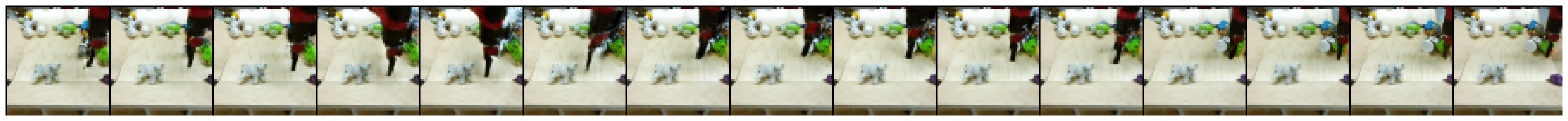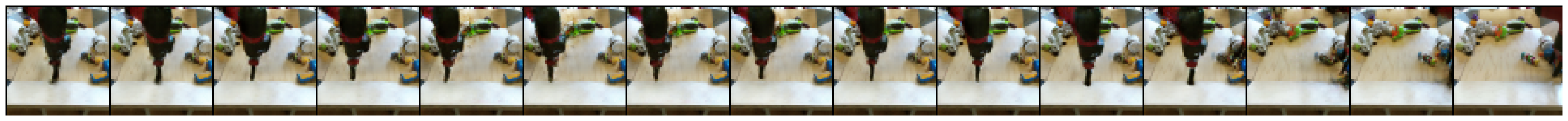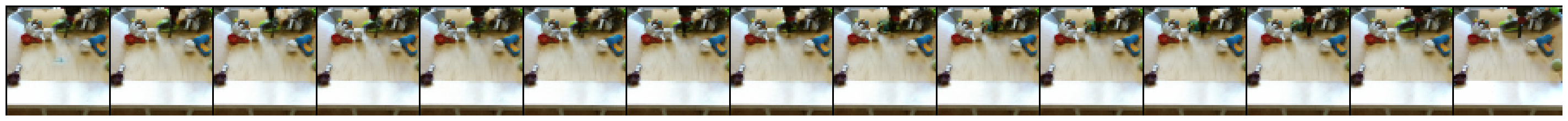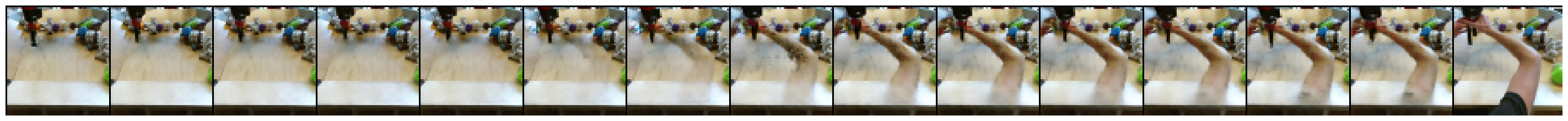[{ "type": "thumb-down", "id": "missingTheInformationINeed", "label":"Missing the information I need" },{ "type": "thumb-down", "id": "tooComplicatedTooManySteps", "label":"Too complicated / too many steps" },{ "type": "thumb-down", "id": "outOfDate", "label":"Out of date" },{ "type": "thumb-down", "id": "translationIssue", "label":"Translation issue" },{ "type": "thumb-down", "id": "samplesCodeIssue", "label":"Samples / code issue" },{ "type": "thumb-down", "id": "otherDown", "label":"Other" }]
[{ "type": "thumb-up", "id": "easyToUnderstand", "label":"Easy to understand" },{ "type": "thumb-up", "id": "solvedMyProblem", "label":"Solved my problem" },{ "type": "thumb-up", "id": "otherUp", "label":"Other" }]